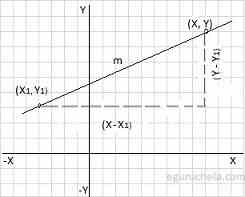### Point slope form Calculator

To calculate equation of a straight line, please enter the line coordinates (x1, y1) in the XY plane are used and Enter Slope Value (m).
The point slope form is defined that the difference in the y-axis coordinate between two points (y - y1) on a line is proportional to the difference in the x-axis coordinate points (x - x1) and the proportionality constant m is the point slope of the straight line. The equation of a straight line can be found using following formula:y – y1 = m(x – x1)
Calculate Equation of a straight line :
 Coordinates X1: Y1: Slope(m): Equation :
Let's take an example where slop is 5 and coordinates (x, y) are (-6 , 8).
Now we can apply these values in given formula as follows:
y – y1 = m(x – x1)
=> y - 8 = 5(x - (-6))
=> y - 8 = 5(x + 6)
=> y - 8 = 5x + 30
=> 5x + 30 -y + 8
= 5x - y + 38 (is the line equation)